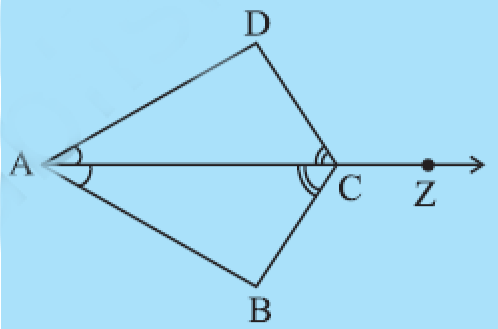Q

# In Fig 7.28, ray AZ bisects angle DAB as well as angle DCB.

5. In Fig 7.28, ray AZ bisects  as well as .

(i) State the three pairs of equal parts in triangles  and .
(ii) Is  Give reasons.
(iv) Is  Give reasons.Views

i)

Given in triangles  and

( common side)

ii)

So, By ASA congruency criterion,triangles  and  are congruent.

iii)

Since  , all corresponding parts will be equal. So

.

iv)

Since  , all corresponding parts will be equal. So

Exams
Articles
Questions